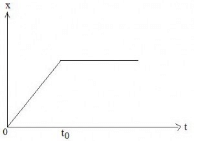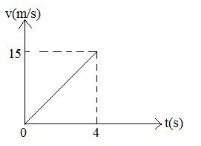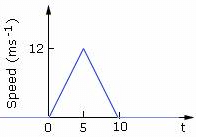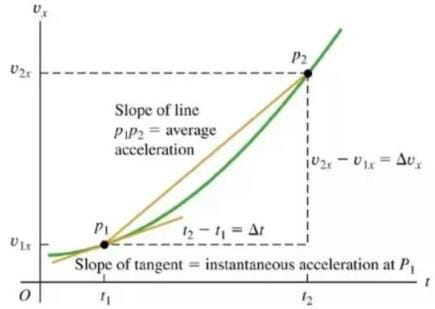NEET  >  Test: Graphical Representation

# Test: Graphical Representation

Test Description

## 10 Questions MCQ Test Physics Class 11 | Test: Graphical Representation

Test: Graphical Representation for NEET 2023 is part of Physics Class 11 preparation. The Test: Graphical Representation questions and answers have been prepared according to the NEET exam syllabus.The Test: Graphical Representation MCQs are made for NEET 2023 Exam. Find important definitions, questions, notes, meanings, examples, exercises, MCQs and online tests for Test: Graphical Representation below.
Solutions of Test: Graphical Representation questions in English are available as part of our Physics Class 11 for NEET & Test: Graphical Representation solutions in Hindi for Physics Class 11 course. Download more important topics, notes, lectures and mock test series for NEET Exam by signing up for free. Attempt Test: Graphical Representation | 10 questions in 10 minutes | Mock test for NEET preparation | Free important questions MCQ to study Physics Class 11 for NEET Exam | Download free PDF with solutions
 1 Crore+ students have signed up on EduRev. Have you?
Test: Graphical Representation - Question 1

### Figure shows the displacement – time graph of a particle moving along X – axis.Detailed Solution for Test: Graphical Representation - Question 1

Because velocity is the slope of the x-t graph.(dx/dt=V) and by looking at the graph it can be seen that upto time T0 slope is constant so velocity will be constant and after time T0 slope is zero ( line is parallel to X axis) so the velocity will be zero.

Test: Graphical Representation - Question 2

### The graph represents the velocity time for the first 4 seconds of motion. The distance covered isDetailed Solution for Test: Graphical Representation - Question 2

Calculate the area of the graph as area of v-t grph gives dis. 1/2×4×15=30

Test: Graphical Representation - Question 3

### A person goes from his home to his office taking one hour. There he works for 2 hours and then in half hour reaches home back. A representative displacement-time graph

Detailed Solution for Test: Graphical Representation - Question 3

We have given in the information that the person is in his office for two hours hence his position must not vary for two hours, that is the horizontal part in the graph which is only observed in the graph of option c.

Test: Graphical Representation - Question 4

Speed time graph of a particle moving along a fixed direction is shown in the figure below. The average speed of the particle over the interval: t = 0 s to 10 s.Detailed Solution for Test: Graphical Representation - Question 4

From the given graph we get that the area of the given graph is total distance covered that is
=  ½ x 10 x 12
=  60m
And total time taken is 10 sec
Thus average speed is  60m / 10sec
= 6 m/s

Test: Graphical Representation - Question 5

The acceleration at any instant is the slope of the tangent of the ________ curve at that instant:

Detailed Solution for Test: Graphical Representation - Question 5

This can be verified graphically

ObtaIning instantaneous acceleration from graphTest: Graphical Representation - Question 6

Slope of displacement time graph or x-t graph gives us the particles’ ____________.

Detailed Solution for Test: Graphical Representation - Question 6

Velocity is a physical vector quantity; both magnitude and direction are needed to define it. The scalar absolute value (magnitude) of velocity is called "speed", being a coherent derived unit whose quantity is measured in the SI (metric system) as metres per second (m/s) or as the SI base unit of (m⋅s−1).

Test: Graphical Representation - Question 7

Two balls of equal mass and of Perfectly elastic material are lying on the floor. One of the balls with velocity V is made to strike the second ball. Both the balls after impact will move with a velocity

Detailed Solution for Test: Graphical Representation - Question 7

Given:

Let two balls A and B have mass mA, mB respectively, and their initial velocities are uA and uB. After the collision, they will move with the same velocity, vo

Given that mass of both balls are same.

So  mA = mB = m

uA = V,  uB = 0

From the Concept of Momentum Conservation:

mAuA + mBuB = (m+m)vo

mV = 2mvo

vo = V/2

Both the balls after impact will move with velocity v/2.

Test: Graphical Representation - Question 8

On velocity-time graph when two curves coincide, the objects will have

Detailed Solution for Test: Graphical Representation - Question 8

A velocity time graph represents the velocity of an object at a very certain time. If two curves collide that means that their velocities at the given time are equal. Their acceleration may or may not be equal depending upon the slope of the curve at the point of intersection.

Test: Graphical Representation - Question 9

Which position – time graph represents the motion of two objects that will never meet?

Detailed Solution for Test: Graphical Representation - Question 9

In the given all options we can see that curves in graph A and D meet in the first quadrant while the curve in option b meets in the second quadrant but those of option c are parallel and hence they never meet.

Test: Graphical Representation - Question 10

Linear inequalities are graphically represented on Cartesian plane by a

Detailed Solution for Test: Graphical Representation - Question 10

The graph of an inequality in two variables is the set of points that represents all solutions to the inequality. A linear inequality divides the coordinate plane into two halves by a boundary line where one half represents the solutions of the inequality. The boundary line is dashed for > and < and solid for ≤ and ≥.

## Physics Class 11

127 videos|464 docs|210 tests
 Use Code STAYHOME200 and get INR 200 additional OFF Use Coupon Code
Information about Test: Graphical Representation Page
In this test you can find the Exam questions for Test: Graphical Representation solved & explained in the simplest way possible. Besides giving Questions and answers for Test: Graphical Representation, EduRev gives you an ample number of Online tests for practice

## Physics Class 11

127 videos|464 docs|210 tests

### How to Prepare for NEET

Read our guide to prepare for NEET which is created by Toppers & the best Teachers